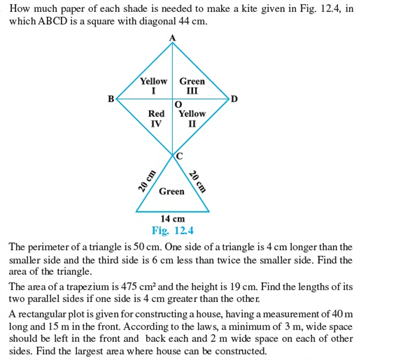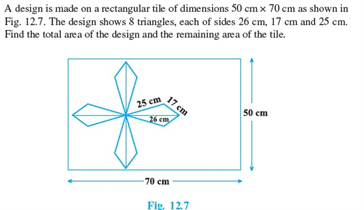# Revision Notes For CBSE Class 9 Math Chapter -12 Heron’s Formula

A formula, which is used to find the surface area of a triangle and any other shape of a triangle, when the lengths of its three sides are known. It can be applied to, as long as we know its three side lengths. The Heron’s formula is given as:

A = √s (s – a) (s – b) ( s – c)

where s = perimeter of a triangle = (a + b + c) / 2

The Heron’s formula was discovered between 10 – 70 AD by a Greek Engineer and Mathematician named Heron of Alexandria. Hence this formula has been named after the name of the discoverer.

Check here for the free CBSE Class 9 Math revision notes for Chapter 12- Heron’s Formula: